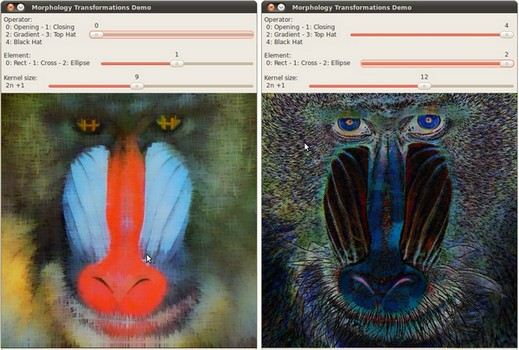OpenCV  4.5.0 Open Source Computer Vision
samples/cpp/tutorial_code/ImgProc/Morphology_2.cppSample screenshot

Check the corresponding tutorial for more details

#include <iostream>
using namespace cv;
Mat src, dst;
int morph_elem = 0;
int morph_size = 0;
int morph_operator = 0;
int const max_operator = 4;
int const max_elem = 2;
int const max_kernel_size = 21;
const char* window_name = "Morphology Transformations Demo";
void Morphology_Operations( int, void* );
int main( int argc, char** argv )
{
CommandLineParser parser( argc, argv, "{@input | baboon.jpg | input image}" );
if (src.empty())
{
std::cout << "Could not open or find the image!\n" << std::endl;
std::cout << "Usage: " << argv << " <Input image>" << std::endl;
return EXIT_FAILURE;
}
namedWindow( window_name, WINDOW_AUTOSIZE ); // Create window
createTrackbar("Operator:\n 0: Opening - 1: Closing \n 2: Gradient - 3: Top Hat \n 4: Black Hat", window_name, &morph_operator, max_operator, Morphology_Operations );
createTrackbar( "Element:\n 0: Rect - 1: Cross - 2: Ellipse", window_name,
&morph_elem, max_elem,
Morphology_Operations );
createTrackbar( "Kernel size:\n 2n +1", window_name,
&morph_size, max_kernel_size,
Morphology_Operations );
Morphology_Operations( 0, 0 );
waitKey(0);
return 0;
}
void Morphology_Operations( int, void* )
{
// Since MORPH_X : 2,3,4,5 and 6
int operation = morph_operator + 2;
Mat element = getStructuringElement( morph_elem, Size( 2*morph_size + 1, 2*morph_size+1 ), Point( morph_size, morph_size ) );
morphologyEx( src, dst, operation, element );
imshow( window_name, dst );
}